# UFR 3-30 Test Case

Jump to navigation Jump to search

# Test Case

## Brief Description of the Test Case Studied

The measures of the geometry introduced by Mellen et al. (2000) relate to the hill height h. The hill constricts the channel height of 3.036h by about one third, whereas the inter hill distance is 9h. The contour of the 3.857h long two-dimensional hill is described by the following six polynomials.

$x\;\epsilon \;\left[0;\;0.3214h\right];\quad y(x)=\min(1;1+0\cdot x+2.420\cdot 10^{-4}\cdot x^{2}-7.588\cdot 10^{-5}\cdot x^{3});$$x\;\epsilon \;\left[0.3215h;\;0.5h\right];\quad y(x)=0.8955+3.484\cdot 10^{-2}\cdot x-3.629\cdot 10^{-3}\cdot x^{2}+6.749\cdot 10^{-5}\cdot x^{3};$$x\;\epsilon \;\left[0.5h;\;0.7143h\right];\quad y(x)=0.9213+2.931\cdot 10^{-2}\cdot x-3.234\cdot 10^{-3}\cdot x^{2}+5.809\cdot 10^{-5}\cdot x^{3};$$x\;\epsilon \;\left[0.7144h;\;1.071h\right];\quad y(x)=1.445-4.927\cdot 10^{-2}\cdot x+6.950\cdot 10^{-4}\cdot x^{2}-7.394\cdot 10^{-6}\cdot x^{3};$$x\;\epsilon \;\left[1.071h;\;1.429h\right];\quad y(x)=0.6401+3.123\cdot 10^{-2}\cdot x-1.988\cdot 10^{-3}\cdot x^{2}+2.242\cdot 10^{-5}\cdot x^{3};$$x\;\epsilon \;\left[1.429h;\;1.929h\right];\quad y(x)=\max(0;2.0139-7.180\cdot 10^{-2}\cdot x+5.875\cdot 10^{-4}\cdot x^{2}+9.553\cdot 10^{-7}\cdot x^{3});$At x/h=0 the hill height is maximal, whereas the boundary is flat in the range between x/h=1.929 and x/h=7.071. Between x/h=7.071 and x/h=9 the contour follows the above equations but the hill geometry is mirrored at x/h = 4.5. Besides the geometry, the following figure shows streamlines at a Reynolds number, that is based on the hill height h and the bulk velocity above the crest, of 5,600 [Rapp 2009)].

$Re={\frac {u_{b}h}{\nu }}$$u_{b}={\frac {1}{2.035}}\int _{h}^{3.035h}u\left(y\right)dy$The mean flow separates at the curved hill crown. In the wake of the hill the fluid recirculates before it attaches naturally at about x/h=4.5.

## Experimental investigation

### Brief description of the experimental setup

The experiments are described in detail in Rapp (2009). An all in all 11 m long and 90 cm broad water channel has been set up at in the Laboratory for Hydromechanics of the Technische Universität München to investigate the flow experimentally. The materials that were used are mainly PVC, plexiglass and glass to access the flow optically. The hills were cast from monolithic polyurethan blocks. Their surface roughness was ≤1µm. The joints between hills and PVC panels were seamlessly filled with epoxy resin.

In total 10 hills with a height of 50 mm were built into the rectangular channel to accomplish periodicity whilst the measurement range lies between hills seven and eight. To achieve homogeneity in the spanwise direction the width of the channel was 18 hill heights. Emphasis was put on the design of the inlet reservoir to assure a homogeneous inflow into the channel. Flow straighteners (D=0.44h, L=10h≥20d) were embedded in the all in all 40h long inlet zone. A 34h long rectangular section lies between the tenth hill and the outlet reservoir. The following figure sketches the experimental setup.
The water was filtered, decalcified and chlorinated such that it was particle free. Later hollow glass spheres with diameters ranging between 8 µm and 12 µm were chosen as particles for PIV and LDA measurements. The maximum Stokes number $St=t_{r}/t_{\eta }$with the relaxation time tr and the Kolmogorov time tη for the flow is yielded at the highest Reynolds number (37,000) but is still considerably smaller than one; hence the particles can be expected to follow the flow perfectly. The water temperature was permanently recorded and with it the effect on the viscosity and therefore the Reynolds number.

Pressure measurements with high resolution cells (10mbar) were conducted to control the periodicity and the homogeneity of the flow. 2D-PIV field measurements were performed at six different camera locations to ensure a satisfactory resolution and to avoid laser light reflections by placing the camera in the particular optical axis (190mJ Nd:YAG laser, 532nm; 4MPx CCD camera). The PIV results were checked with 1D-LDA point measurements (75mW Nd:YAG laser, 532nm). The LDA measurements were undertaken at 20 vertical positions at x/h=0.05 (close to the point of seperation) and 29 at x/h=4.0 (close to the point of reattachment). The averaging of the approx. 40,000 LDA samples per measurement point was done by time integration according to Barnett (1974). 10,000 PIV samples were taken for each experiment. The partly overlapping interrogation areas resolved approx 0.09h.

The measurements were undertaken between hills seven and eight - and to investigate the periodicity of the flow - between the hill pair six and seven. The homogeneity in the spanwise direction was controlled by 2D PIV measurements in horizontal planes. Experiments were done at four Reynolds numbers: Re=5,600; Re=10,600; Re=19,000 and Re=37,000. The quantities that are presented are
the average streamwise velocity component $\langle u\rangle /u_{b}$,
the average vertical velocity component $\langle v/u_{b}\rangle$,
the average normal stress in the x direction $\langle u\prime u\prime \rangle /u_{b}^{2}$,
the average normal stress in the y direction $\langle v\prime v\prime \rangle /u_{b}^{2}$and the
the average shear stress $\langle u\prime v\prime \rangle /u_{b}^{2}$.

### Experimental data available

The data are available for the four Reynolds numbers at the x/h positions x/h=0.05, x/h=0.5, x/h=1.0, x/h=2.0, x/h=3.0, x/h=4.0, x/h=5.0, x/h=6.0, x/h=7.0, x/h=8.0.

Experiment Re=5600    x/h=0.05, x/h=0.5, x/h=1.0, x/h=2.0, x/h=3.0, x/h=4.0, x/h=5.0, x/h=6.0, x/h=7.0, x/h=8.0

Experiment Re=10,600 x/h=0.05, x/h=0.5, x/h=1.0, x/h=2.0, x/h=3.0, x/h=4.0, x/h=5.0, x/h=6.0, x/h=7.0, x/h=8.0

Experiment Re=19,000 x/h=0.05, x/h=0.5, x/h=1.0, x/h=2.0, x/h=3.0, x/h=4.0, x/h=5.0, x/h=6.0, x/h=7.0, x/h=8.0

Experiment Re=37,000 x/h=0.05, x/h=0.5, x/h=1.0, x/h=2.0, x/h=3.0, x/h=4.0, x/h=5.0, x/h=6.0, x/h=7.0, x/h=8.0

## CFD Methods

The numerical part of the present study relies on two completely independent codes based on either curvilinear body-fitted grids with a colocated variable arrangement or Cartesian non-uniform grids using a staggered configuration. The objective is to present highly reliable results obtained by carefully cross-checking between the outcome of both numerical schemes and additional experimental data. Afterwards the investigations concentrate on the physical aspects of the flow considered.

In the following, both codes are described briefly. Information on the separate calculations performed in the ATAAC project is given in D3.2-36_Jakirlic-ST01-ERCOFTAC-WIKI.pdf.

### Finite-volume code LESOCC

LESOCC solves the (filtered) Navier-Stokes equations based on a three-dimensional finite-volume method for arbitrary non-orthogonal and non-staggered block-structured grids (see Fig. 3.4). The spatial discretization of all fluxes is based on central differences of second-order accuracy. Time advancement is performed by a predictor--corrector scheme. A low-storage multi-stage Runge-Kutta method (three sub-steps, second-order accuracy) is applied for integrating the momentum equations in the predictor step. Within the corrector step the Poisson equation for the pressure correction is solved implicitly by the incomplete LU decomposition method. Explicit time marching works well for DNS and LES with small time steps which are necessary to resolve turbulence motion in time. In order to ensure the coupling of pressure and velocity fields on non-staggered grids, the momentum interpolation technique is used. For modeling the non-resolvable subgrid scales, two different models are implemented, namely the well-known Smagorinsky model (1963) with Van Driest damping near solid walls and the dynamic approach with a Smagorinsky base model proposed by Germano et al. (1991) and modified by Lilly (1992). In order to stabilize the dynamic model, averaging of the numerator and the denominator in the relation for the determination of the Smagorinsky value was carried out in the spanwise homogeneous direction and also in time using a recursive digital low-pass filter (Breuer and Rodi 1996, Breuer 2002). The code and the implemented SGS models were validated on a variety of different test cases. For more information on this issue, please refer to Breuer and Rodi (1996), Breuer (1998, 2000, 2002).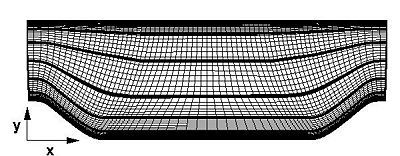Fig. 3.4 Cross-section (x-y) of the curvilinear grid; every fourth grid line is shown; thick lines depict block boundaries

### Finite-volume code MGLET

MGLET is based on a finite-volume formulation for non-uniform Cartesian grids with a staggered arrangement of the spatially filtered variables (see Fig. 3.5). The spatial discretization of the convective and diffusive fluxes is based on second-order central differences. The momentum equations are advanced in time by a fractional time stepping using either an explicit second-order central leapfrog scheme or a third-order Runge-Kutta scheme. For the solution of the Poisson equation for the pressure the Strongly Implicit Procedure (SIP) is implemented. For the representation of the hill geometry in the Cartesian grid an immersed boundary technique is used. All Cartesian cells lying inside the body are excluded from the computation. The excluded cells are determined by the intersection of the hill geometry with the Cartesian cells. The geometry of the hills is represented by a triangle mesh. The immersed boundary technique provides a smooth representation of the body surface in the Cartesian mesh by using third-order least squares interpolation for the interface cells (Peller 2006). This method prevents instabilities which are present in high-order Lagrange interpolation schemes. The code is used for DNS and LES simulations. It has been shown by several authors that second-order accuracy can be sufficient for DNS of flows provided the grid resolution is sufficient (Manhart and Friedrich 2002, Peller et al. 2006).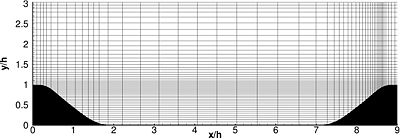Fig. 3.5 Cross-section (x-y) of the Cartesian grid; every 15th grid line is shown

### Boundary conditions and simulation parameters

Since the grid resolution in the vicinity of the wall is sufficient to resolve the viscous sublayer, the no-slip and impermeability boundary condition is used at the wall in both codes. The flow is assumed to be periodic in the streamwise direction and thus periodic boundary conditions are applied. Similar to the turbulent plane channel flow case the non-periodic behavior of the pressure distribution can be accounted for by adding the mean pressure gradient as a source term to the momentum equation in streamwise direction. Two alternatives exist. Either the pressure gradient is fixed which might lead to an unintentional mass flux in the configuration or the mass flux is kept constant which requires to adjust the mean pressure gradient in time. Since a fixed Reynolds number can only be guaranteed by a fixed mass flux, the second option is chosen here.

Furthermore, the flow is assumed to be homogeneous in spanwise direction and periodic boundary conditions are applied, too. For that purpose the use of an adequate domain size in the spanwise direction is of major importance in order to obtain reliable and physically reasonable results. To assure this criterion the two-point correlations in the spanwise direction have to vanish in the half-width of the domain size chosen. Based on the investigations by Mellen et al. (2000) a spanwise extension of the computational domain of $L_{z}=4.5h$is used in all computations presented. This extension of the domain was also confirmed and used in the investigation by Fröhlich et al. (2005). It represents a well-balanced compromise between spanwise extension and spanwise resolution.

Table 1 summarizes the most important parameters of the simulations available. $N_{tot}$denotes the total number of grid points used; the corresponding number of control volumes is slightly lower. Although a direct comparison of the number of grid points used by LESOCC and MGLET in one x-y plane is not reasonable, at least the number of points equidistantly distributed in the spanwise direction, $N_{span}$, can be compared.

The dimensionless time-step size $\Delta t$is also tabulated in Table 1, where the time is normalized by the ratio of the hill height h and the bulk velocity ${U_{B}}$taken at the crest of the hill. To reduce statistical errors due to insufficient sampling to a reasonable minimum, the flow field was averaged in spanwise direction and in time over a long period of $\Delta T_{avg}$which is also given in Table 1. Partially $\Delta T_{avg}$covers a time interval of about ${140}$flow-through times.

Table 1: Parameters of the simulations performed including Reynolds number, simulation type (DNS/LES), total number of grid points, number of grid points in spanwise direction, dimensionless time-step size, dimensionless averaging period, and code applied.

Case Re Sim. Ntot/106 Nspan Δt/10-3 Tavg Code
1 700 DNS 13.1 200 0.5 1581 LESOCC
2 1400 DNS 13.1 200 1.1 1249 LESOCC
3 1400 DNS 20.0 132 1.5 540 MGLET
4 2800 DNS 13.1 200 2.0 1249 LESOCC
5 2800 DNS 48.0 304 1.0 562 MGLET
6 5600 DNS 13.1 200 2.0 1214 LESOCC
7 5600 LES 13.1 200 2.0 1303 LESOCC
8 5600 DNS 231.0 404 1.0 343 MGLET
9 10,595 LES 13.1 200 1.8 1277 LESOCC
10 10,595 LES 4.1 104 1.0 241 MGLET
11 37,000 LES 4.1 104 1.0 439 MGLET

### Resolution issues

A detailed discussion about this concern is provided in Breuer et al. (2009). Here only a few issues should be mentioned. We start with the curvilinear grid design for the wall-resolved LES prediction at the highest Reynolds number chosen, i.e. Re = 10,595.

#### Curvilinear grids

For the simulation at Re = 10,595 LESOCC applies a curvilinear block-structured grid consisting of $N_{tot}=13.1$million grid points corresponding to a total of about 12.4 million control volumes. The grid points are clustered in the vicinity of the lower wall, the upper wall, and the region where the free shear layer appears. Besides classical quality criteria such as orthogonality and smoothness, two main issues motivated the distribution of the grid points in space. These are the resolution of the near-wall region and of the inner domain.

To evaluate the first concern, the most important quality criterion is the distribution of non-dimensional $y^{+}$values defined by $y^{+}=\Delta y_{cc}\,u_{\tau }/\nu$where $\Delta y_{cc}$denotes the distance of the cell center from the wall and $u_{\tau }={\sqrt {\tau _{w}/\rho }}$describes the shear stress velocity. Note that due to the cell-centered variable arrangement $\Delta y_{cc}$is half of the corresponding cell height $\Delta y$. The following figure depicts the $y^{+}$distribution along the lower wall at all Reynolds numbers considered. At Re = 10,595 the values are below 0.45 with a mean value of about 0.2 except at the windward side of the hill. Here the largest values of the wall shear stress are observed and the $y^{+}$value reaches its maximum of about 1.2. Hence the lower wall is well resolved. Regarding the wall-normal resolution the grid satisfies the requirements of a wall-resolved LES prediction. Compared to Fröhlich et al (2005) who employed in their highly resolved simulations a curvilinear grid with about 4.6 million CVs (196 x 128 x 186) especially the number of grid points in the wall-normal direction was increased to 220 in the present investigation. Furthermore, the simulations resolve not only the lower wall (the hills) in more detail but also resolve the upper wall by a DNS-like representation ($y^{+}\leq 0.95$at Re = 10,595). Thus in contrast to Fröhlich et al (2005) the application of wall functions is avoided. That allows to establish the influence of the resolution of the upper wall on the results. The results are displayed in the evaluation section below.

To prove the enhanced resolution, some numbers are provided. For instance, the cell sizes at the hill crest, which is a key region for the periodic hill flow are in the current case $\Delta x_{crest}/h=0.026$and $\Delta y_{crest}/h=2.0\times 10^{-3}$whereas the corresponding values in Fröhlich et al (2005) are $\Delta x_{crest}/h=0.032$and $\Delta y_{crest}/h=3.3\times 10^{-3}$, respectively. Owing to the increased resolution in streamwise and spanwise direction the cell sizes expressed in wall units are below $\Delta x^{+}=20$and $\Delta z^{+}=9$and thus lower than in Fröhlich et al (2005) and substantially lower than the recommendations for wall-resolved LES. That also holds at the windward slope of the hill where the largest shear stresses are found.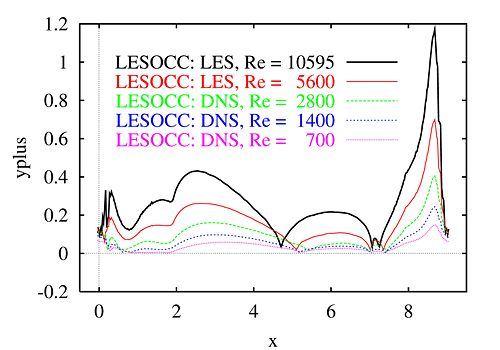Fig. 3.6 Distribution of $y^{+}$along the lower wall at different Re using LESOCC

To evaluate the second concern, the resolution of the inner region, it is reasonable to estimate the size of the smallest scales given by the Kolmogorov length $\eta =(\nu ^{3}/\epsilon )^{1/4}$. In order to determine this quantity within the wall-resolved LES prediction, the dissipation tensor $\epsilon _{ij}$was predicted and averaged in time and in spanwise direction. Based on this procedure it was found that the grid allows to resolve a substantial part of the dissipation, see Breuer et al. (2009) for all details.

The generation of the grid for LESOCC was designed for the wall-resolved LES predictions at Re = 10,595. Regarding the lower Reynolds numbers considered, the grid was not modified when Re was reduced. The reason is twofold. On the one hand a grid which is sufficiently fine for a certain Reynolds number should also be adequate for a lower Re. That is visible for example in the figure depicted above which depicts the distributions of $y^{+}$along the lower wall for all Re. The shear stress increases with decreasing Reynolds numbers, but for fixed $U_{B}$and h the viscosity also increases with decreasing Re. As a result the average and maximum $y^{+}$values are strongly reduced. Consequently, with decreasing Re the resolution becomes better and better. Thus by applying the same grid for the comparison of two Re, the effect of the grid is completely excluded from the consideration. On the other hand the intention was to perform DNS predictions for the lowest Reynolds numbers considered, i.e. at Re = 700, 1400, and 2800. Consequently, the grid should be sufficiently fine for a DNS at Re = 2800. That is exactly the Reynolds number in the classical plane channel flow predictions by Moser et al. (1999) who applied a grid of about 2 million points. In the present case a six times finer grid is applied which accounts for the more complex flow field and the lower accuracy of the numerical method. Besides the criteria discussed in detail above, a further evidence of the adequacy of the grid for DNS at $Re\leq 2800$is provided by two simulations carried out at Re = 5600 (see Table 1). One simulation was done as a wall-resolved LES using the dynamic SGS model (case 7) and the other was carried out without a subgrid-scale model (case 6). As will be discussed below, only marginal deviations were found between both cases. That is a clear hint that for the further reduced Reynolds numbers $Re\leq 2800$the grid used delivers results which can be regarded as DNS.

The simulation at Re = 10,595 (case 9) was performed with the dynamic Smagorinsky model. Owing to the increased resolution the ratio of $\nu _{t}/\nu$found in the present prediction is smaller than in the previous study. Moreover, by applying two different SGS models which delivered strongly differing eddy-viscosity values, Fröhlich et al. (2005) have shown that the influence of this deviation on the LES prediction is low if a very fine grid is used as in the present case. That confirms that the present simulation is not materially inferior to a DNS near the walls.

#### Cartesian grids

The most important issue for the Cartesian grid used by MGLET are the Kolmogorov scale in the domain and the resolution at the wall. For the immersed boundary method further criteria were defined. A preliminary evaluation of these criteria has been obtained by simulations with relatively coarse grids. The resolution estimations were confirmed by the finally computed values [Peller and Manhart (2006)]. As strong gradients must be resolved close to the hill contour the grid was refined for the DNS at Re=2800 (case 5) in the y direction. Anyway, the stretching factors are kept under 3%. The grid is refined in the y direction and therefore not exactly wall normal at all positions of the geometry. The grid is equidistant in the streamwise and the spanwise direction. For the DNS at Re=5600 (case 8) the mesh was also refined in the x direction. Special emphasis has been put on controlling the spacial resolution as for Cartesian grids small grid spacings lead to a large number of grid points, also in regions where it is not needed. It is therefore important to reduce the number of grid points by stretching at positions with low wall shear stress and condensing the number of grid points at locations with high wall shear stress. The inner domain is well resolved (Δ/η≤2.2) and the grid refinement compensates for the decrease in Kolmogorov length scale close to the wall. For the grid generation for DNS with immersed boundaries the following four criteria have to be met:

• the absolute wall-normal distance must not exceed d+≈1
• the stretching factor has to be below 3%
• the aspect ratio must not be greater than 9 and
• the ratio of grid spacings and the Kolmogorov length scale have to be small in the entire domain.

Following theses rules the DNS grid for Re=1400 (case 3) yielded 20 million and for Re=5600 (case 8) 231 million grid points.

For the LES at Re=10,595 (case 10)

• the absolute wall-normal distance did not exceed d+≈11
• the stretching factor was below 7% and
• the aspect ratio was smaller than 12.

The same grid was used for the LES at Re=37,000 (case 11).

### CFD data available

#### Curvilinear grids

For the cases 1,2,4,7 and 9 in Table 1 profiles of the computed data are available at the following 10 positions:

 Coordinate x/h = 0.05, 0.5, 1.0, 2.0, 3.0, 4.0, 5.0, 6.0, 7 and 8.0 File: 001, 002, 003, 004, 005, 006, 007, 008, 9 and 010

The data sets include $y/h,\langle U/U_{b}\rangle ,\langle V/U_{b}\rangle ,\langle u\prime u\prime /U_{b}^{2}\rangle ,\langle v\prime v\prime /U_{b}^{2}\rangle ,\langle u\prime v\prime /U_{b}^{2}\rangle ,\langle k/U_{b}^{2}\rangle$.

Case 1 DNS (LESOCC) Re = 700      001 002 003 004 005 006 007 008 009 010

Case 2 DNS (LESOCC) Re = 1400    001 002 003 004 005 006 007 008 009 010

Case 4 DNS (LESOCC) Re = 2800    001 002 003 004 005 006 007 008 009 010

Case 7 DNS (LESOCC) Re = 5600    001 002 003 004 005 006 007 008 009 010

Case 9 LES (LESOCC) Re = 10,595  001 002 003 004 005 006 007 008 009 010

Exemplarly, some profiles at x/h = 2 and 4 are depicted for five different Reynolds numbers.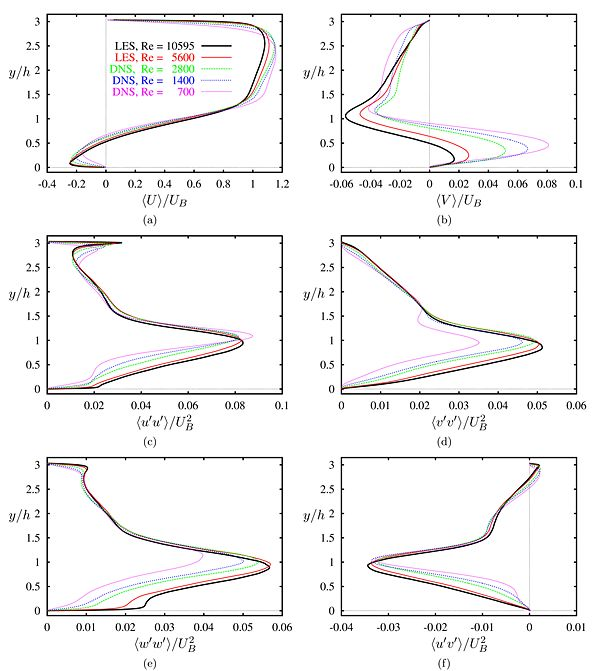Fig. 3.7 Profiles of the streamwise velocity, the normal velocity, and the resolved Reynolds stresses at position x/h = 2.0 for five different Reynolds numbers, predictions by LESOCC.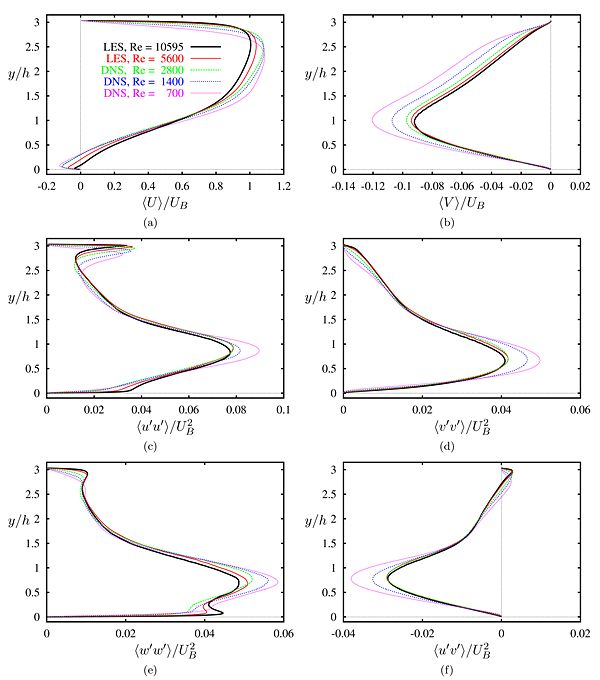Fig. 3.8 Profiles of the streamwise velocity, the normal velocity, and the resolved Reynolds stresses at position x/h = 4.0 for five different Reynolds numbers, predictions by LESOCC.

#### Results from ATAAC project

Results from the ATAAC project obtained with RANS and Hybrid LES-RANS methods for the Reynolds numbers 10,595 and 37,000 are given in D3.2-36_Jakirlic-ST01-ERCOFTAC-WIKI.pdf (extended excerpt from an ATAAC report).

Contributed by: (*) Christoph Rapp, (**) Michael Breuer, (*) Michael Manhart, (*) Nikolaus Peller — (*) Technische Universität München, (**) Helmut-Schmidt Universität Hamburg

© copyright ERCOFTAC 2009, 2013